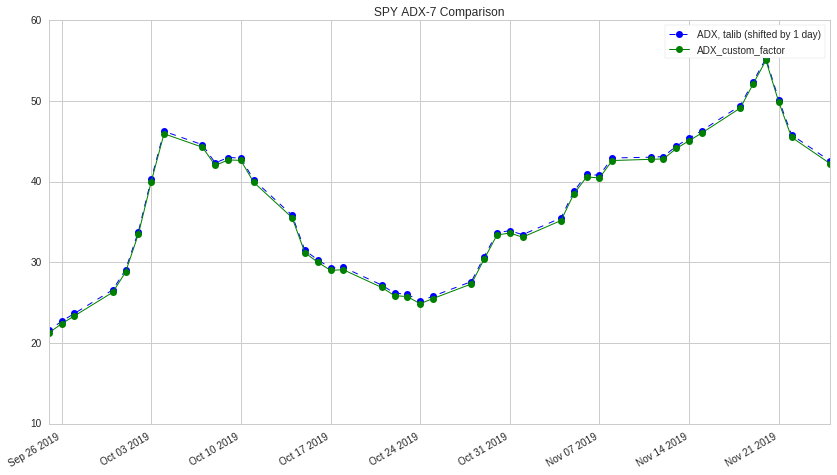Notebook

ADX - Custom factor for pipeline (WIP)

In :
from quantopian.pipeline import Pipeline
from quantopian.pipeline.data.builtin import USEquityPricing
from quantopian.pipeline.filters import StaticAssets
from quantopian.pipeline.factors import CustomFactor
from quantopian.research import run_pipeline

import numpy as np

inputs = [USEquityPricing.high, USEquityPricing.low, USEquityPricing.close]
window_length = 140            # use window_length = 10 * adx_len

def compute(self, today, assets, out, highs, lows, closes, adx_len):
THs = np.maximum(highs[1:], closes[:-1])  # max of current high or previous close
TLs = np.minimum(lows[1:], closes[:-1])   # min of current low or previous close
TRs = THs - TLs

# This comented out code below is an efficient way to calculate ATR without a for loop
# However it produces a single ATR value and not the array of ATR values needed later on
#count = len(TRs)
#decay_rate = (1.0 - (1.0 / (adx_len)))
#weights = np.full(count, decay_rate, float) ** np.arange(count + 1, 1, -1)
#ATR = np.average(TRs, axis=0, weights=weights)

ATRs = np.empty(TRs.shape)
ATRs.fill(np.nan)

for j in range(0, TRs.shape):
else:

high_diffs = highs[1:]-highs[:-1]  # current high - previous high
low_diffs = lows[:-1]-lows[1:]     # previous low  - current low

pDIs = np.where(((high_diffs > low_diffs) & (high_diffs > 0)), high_diffs, 0.)
nDIs = np.where(((low_diffs > high_diffs) & (low_diffs > 0)), low_diffs, 0.)

# This comented out code below is an efficient way to calculate ApDI and AnDI without a for loop
# However it produces single values and not the array of values needed later on
#ApDI = np.average(pDIs, axis=0, weights=weights)
#AnDI = np.average(nDIs, axis=0, weights=weights)

ApDIs = np.empty(pDIs.shape)
ApDIs.fill(np.nan)

for j in range(0, pDIs.shape):
else:

AnDIs = np.empty(nDIs.shape)
AnDIs.fill(np.nan)

for j in range(0, nDIs.shape):
else:

pDMs = 100 * (ApDIs / ATRs)
nDMs = 100 * (AnDIs / ATRs)

DXs = 100 * np.abs(pDMs - nDMs) / (pDMs + nDMs)

# This commented out code below seems like it should have worked
# However it just produce NaN values.
#count = len(DXs)
#decay_rate = (1.0 - (1.0 / (adx_len)))
#weights = np.full(count, decay_rate, float) ** np.arange(count + 1, 1, -1)

for j in range(0, DXs.shape):
else:

# Uncomment out[:] statements below to check intermediate calculations
# for example, create excel ADX spreadsheet and check corresponding column calculations

#out[:] = highs[-1]
#out[:] = lows[-1]
#out[:] = closes[-1]
#out[:] = THs[-1]
#out[:] = TLs[-1]
#out[:] = TRs[-1]
#out[:] = TRs.shape
#out[:] = ATRs[-1]
#out[:] = ATRs.shape
#out[:] = high_diffs[-1]
#out[:] = low_diffs[-1]
#out[:] = pDIs[-1]
#out[:] = pDIs.shape
#out[:] = pDIs.shape
#out[:] = nDIs[-1]
#out[:] = nDIs.shape
#out[:] = nDIs.shape
#out[:] = ApDIs[-1]
#out[:] = ApDIs.shape
#out[:] = ApDIs.shape
#out[:] = AnDIs[-1]
#out[:] = pDMs[-1]
#out[:] = pDMs.shape
#out[:] = pDMs.shape
#out[:] = nDMs[-1]
#out[:] = DXs[-1]
#out[:] = DXs.shape
#out[:] = DXs.shape

# problem - The for loops used for Wilder smoothing of ATR, +DI, -DI, and ADX are very slow

In :
def create_pipeline():

# Base universe set to one symbol for easy validation
base_universe = StaticAssets(symbols(['spy']))

# Uncomment the line below to test if it works for multple symbols
#base_universe = StaticAssets(symbols(['spy', 'tlt']))

# Factor of yesterday's close price.
yesterday_close = USEquityPricing.close.latest

# Factor of yesterday's ADX with default length of 7.

pipe = Pipeline(
columns={
'yesterday_close': yesterday_close,
},
screen=base_universe
)
return pipe

results = run_pipeline(create_pipeline(), '11-26-2018', '11-25-2019')

import pandas as pd
results.index = pd.MultiIndex.droplevel(results.index, level=1)
results.tail(20)


Pipeline Execution Time: 6.94 Seconds
Out:
2019-10-29 00:00:00+00:00 30.411124 303.36
2019-10-30 00:00:00+00:00 33.393022 303.20
2019-10-31 00:00:00+00:00 33.638675 304.14
2019-11-01 00:00:00+00:00 33.122921 303.26
2019-11-04 00:00:00+00:00 35.189766 306.18
2019-11-05 00:00:00+00:00 38.488025 307.34
2019-11-06 00:00:00+00:00 40.589182 307.01
2019-11-07 00:00:00+00:00 40.475036 307.14
2019-11-08 00:00:00+00:00 42.626389 308.22
2019-11-11 00:00:00+00:00 42.769598 308.96
2019-11-12 00:00:00+00:00 42.828381 308.39
2019-11-13 00:00:00+00:00 44.121558 308.94
2019-11-14 00:00:00+00:00 45.080786 309.07
2019-11-15 00:00:00+00:00 46.048021 309.53
2019-11-18 00:00:00+00:00 49.136464 311.83
2019-11-19 00:00:00+00:00 52.121015 311.99
2019-11-20 00:00:00+00:00 55.049215 311.89
2019-11-21 00:00:00+00:00 49.896148 310.79
2019-11-22 00:00:00+00:00 45.515419 310.29
2019-11-25 00:00:00+00:00 42.259032 311.00
In :
import talib as ta
import matplotlib.pyplot as plt

# Get data for SPY
data = get_pricing('spy', '11-26-2018', '11-25-2019', frequency='daily')

# fields: 'open_price', 'high', 'low', 'close_price'
date = data.index
openp = data['open_price']
closep = data['close_price']
highp = data['high']
lowp = data['low']

# Calculate ADX-7 based on talib
#data = data[1:]

# Shift by one day to align with pipeline and
# offset it vertically by 0.3 so that it is not line on line with ADX from pipeline